### Home > PC > Chapter 1 > Lesson 1.1.2 > Problem1-26

1-26.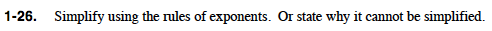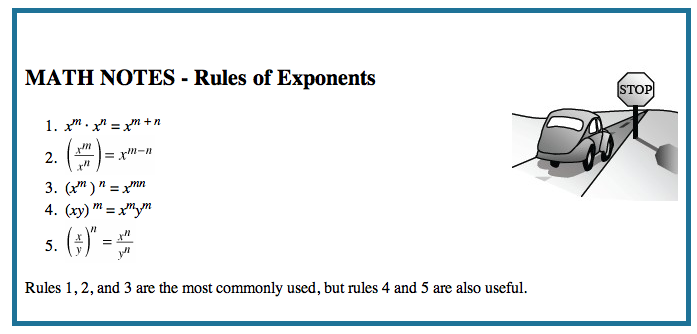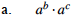$a^{b+c}$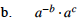$a^{(c-b)}$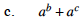Can't be simplified. We are not multiplying.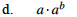$a^{b+1}$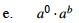$a^b$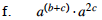$a^{(3c+b)}$